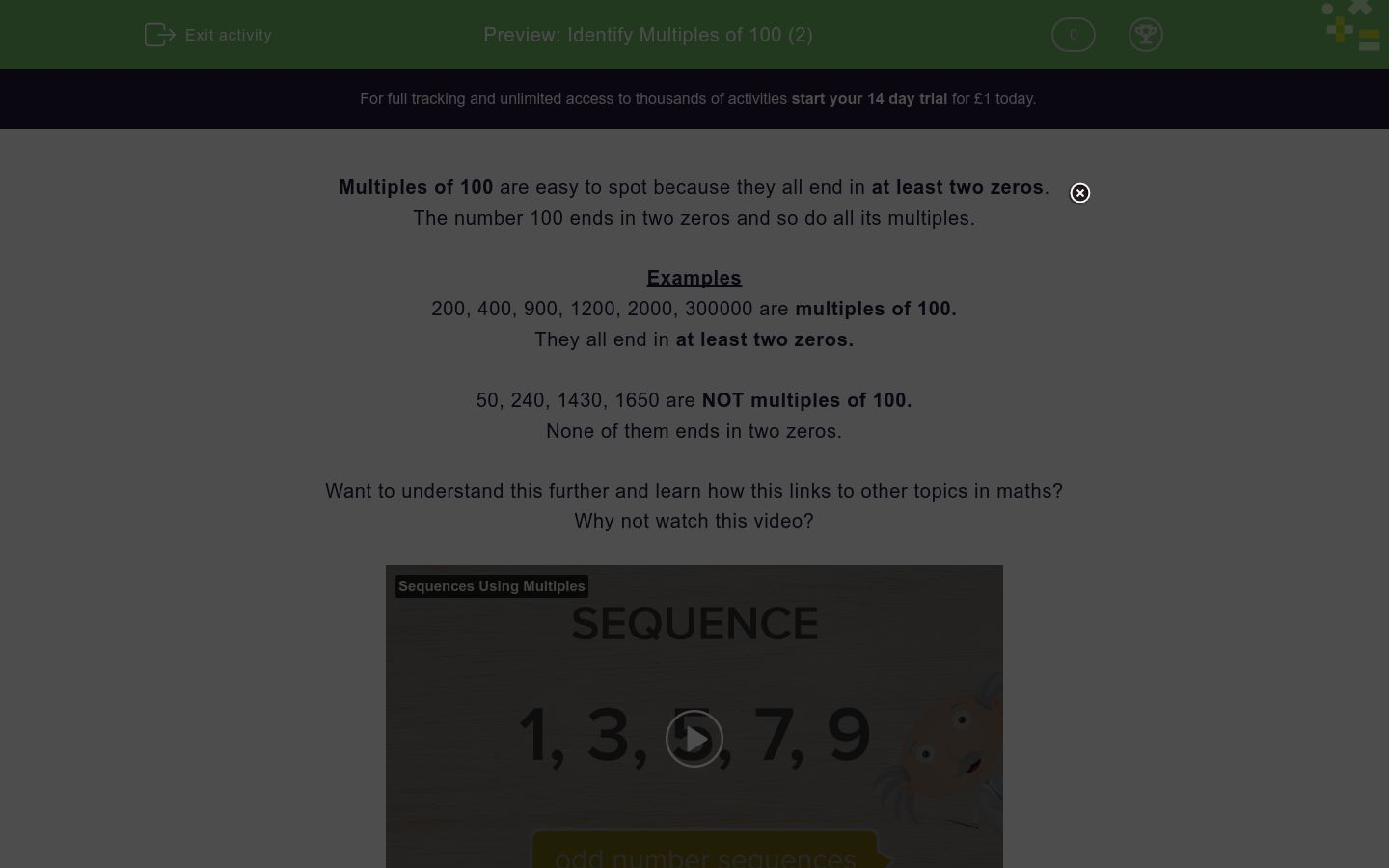# Identify Multiples of 100 (2)

In this worksheet, students answer questions on multiples of 100.Key stage:  KS 2

Curriculum topic:  Number: Number and Place Value

Curriculum subtopic:  Count in Multiples (4, 8, 50 and 100)

Difficulty level:### QUESTION 1 of 10

Multiples of 100 are easy to spot because they all end in at least two zeros.

The number 100 ends in two zeros and so do all its multiples.

Examples

200, 400, 900, 1200, 2000, 300000 are multiples of 100.

They all end in at least two zeros.

50, 240, 1430, 1650 are NOT multiples of 100.

None of them ends in two zeros.

Select all the numbers which are multiples of 100.

833

739

800

1000

500

148

Select all the numbers which are multiples of 100.

385

107

400

500

700

130

Select all the numbers which are multiples of 100.

200

100

20

300

209

458

Select all the numbers which are multiples of 100.

815

500

627

100

200

540

Select all the numbers which are multiples of 100.

1000

521

497

926

100

800

Select all the numbers which are multiples of 100.

702

949

800

85

4000

700

Select all the numbers which are multiples of 100.

498

308

500

400

44

200

Select all the numbers which are multiples of 100.

227

300

936

800

345

400

Select all the numbers which are multiples of 100.

200

800

310

159

942

3000

Select all the numbers which are multiples of 100.

10

20

25

50

100

250

• Question 1

Select all the numbers which are multiples of 100.

CORRECT ANSWER
800
1000
500
EDDIE SAYS
500 and 800 end in two zeros.
1000 ends in three zeros.
• Question 2

Select all the numbers which are multiples of 100.

CORRECT ANSWER
400
500
700
EDDIE SAYS
400, 500 and 700 end in two zeros.
• Question 3

Select all the numbers which are multiples of 100.

CORRECT ANSWER
200
100
300
EDDIE SAYS
100, 200 and 300 end in two zeros.
• Question 4

Select all the numbers which are multiples of 100.

CORRECT ANSWER
500
100
200
EDDIE SAYS
100, 200 and 500 end in two zeros.
• Question 5

Select all the numbers which are multiples of 100.

CORRECT ANSWER
1000
100
800
EDDIE SAYS
100 and 800 end in two zeros.
1000 ends in three zeros.
• Question 6

Select all the numbers which are multiples of 100.

CORRECT ANSWER
800
4000
700
EDDIE SAYS
700 and 800 end in two zeros.
4000 ends in three zeros.
• Question 7

Select all the numbers which are multiples of 100.

CORRECT ANSWER
500
400
200
EDDIE SAYS
200, 400 and 500 end in two zeros.
• Question 8

Select all the numbers which are multiples of 100.

CORRECT ANSWER
300
800
400
EDDIE SAYS
300, 400 and 800 end in two zeros.
• Question 9

Select all the numbers which are multiples of 100.

CORRECT ANSWER
200
800
3000
EDDIE SAYS
200 and 800 end in two zeros.
3000 ends in three zeros.
• Question 10

Select all the numbers which are multiples of 100.

CORRECT ANSWER
100
EDDIE SAYS
100 is the only number listed that ends in two zeros.
---- OR ----

Sign up for a £1 trial so you can track and measure your child's progress on this activity.

### What is EdPlace?

We're your National Curriculum aligned online education content provider helping each child succeed in English, maths and science from year 1 to GCSE. With an EdPlace account you’ll be able to track and measure progress, helping each child achieve their best. We build confidence and attainment by personalising each child’s learning at a level that suits them.

Get startedStart your £1 trial today.
Subscribe from £10/month.